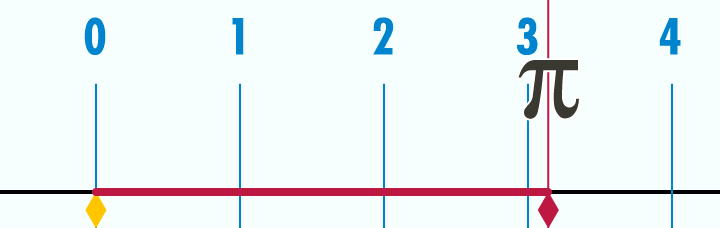# How to Calculate the Circumference of a Circle

In the previous post, we have learned about the basic terminologies about circles. We continue this series by understanding the meaning of circumference of a circle. The circumference of a circle is basically the distance around the circle itself. If you want to find the circumference of a can, for example, you can get a measuring tape and wrap around it.

The animation below shows, the meaning of circumference. As we can see, the circle with diameter 1 has circumference$\pi$ or approximately$3.14$.Note: If you want to know where$\pi$ came from, read Calculating the Value of Pi.

Example 1

What is the perimeter of a circle with diameter 1 unit?

Solution

The formula of finding the circumference of a circle is with circumference$C$ and diameter$d$ is$C = \pi d$. So,$C = \pi d = \pi(1) = \pi$.

Example 2

Find the circumference of a circle with radius 2.5 cm.

Solution

The circumference$C$ of a circle with radius$r$ is$C = 2 \pi r$

So,$C = 2(3.14)(2.5) = 15.7$

Therefore, the circumference of a circle with radius 2.5 cm is 15.7 cm.

Example 3

Find the radius of a circle with a circumference 18.84 cm. Use$\pi = 3.14$.

Solution$C = 2 \pi r$$18.84 = 2 (3.14) r$$18.84 = 6.28 r$

Dividing both sides by 6.28, we have$3 = r$.

Therefore, the radius of a circle with circumference 18.84 cm is 3 cm.

Example 4

Mike was jogging in circular park. Halfway completing the circle, he went back to where he started through a straight path. If he traveled a total distance of 514 meters, what is the total distance if he jogged around the park once? (Use$\pi = 3.14$).

Solution

The distance traveled by Mike is equal to half the circumference of the circular park and its diameter. Since the circumference of a circle is$2 \pi r$ and the diameter is equal to$2r$, the distance traveled by Mike is

So,$D = \frac{1}{2}(2 \pi r) + 2r$.

Substituting, we have$514 = \pi r + 2r$.

Factoring out$r$, we have$514 = r( \pi + 2)$$514 = r(3.14 + 2)$$514 = r (5.14)$.

Dividing both sides by 5.14, we get$r = 100$.

Now, we are looking for the distance around the park (cirumfrence of the circle). That is,$C = 2 \pi r = 2 (3.14)(100)$$C = 628$ meters.

In the next post, we will discuss how to calculate the area of a circle.